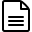# How is CPU Utilization calculated for AIX server?

## CPU Utilization

We use the following methods of calculation for CPU Utilization when lparstat command is supported:

Method 1: If   'type=Dedicated ', then we use the vmstat command output. (i.e) cpu =100-Idle Time
Method 2: If 'type=Shared' , ' mode=UnCapped', and 'smt=On' , then cpu =lcpu/2
Method 3: If  'type=Shared' , ' mode=UnCapped', and  'smt' is disabled or not available , then cpu =lcpu
Method 4: If  'type=Shared' , ' mode=UnCapped', and  'smt=x' , then cpu =lcpu/x
Method 5: If  'type=Shared' , and ' mode=Capped', then cpu =ent

CPU Utilization can be calculated by replacing  cpu (calculated from the above methods) in the below calculation:

CPU Utilization %=(physc*100)/cpu

## CPU Core Utilization

If you have multiple cores, we show individual CPU cores usage calculation we use the below command and this is shown in a different tab (there is a separate CPU tab where this individual cores usage is shown).

Command:  sar -u -P ALL 1 3Note: These calculations are based on the feedback from our customers who are AIX admins/users.